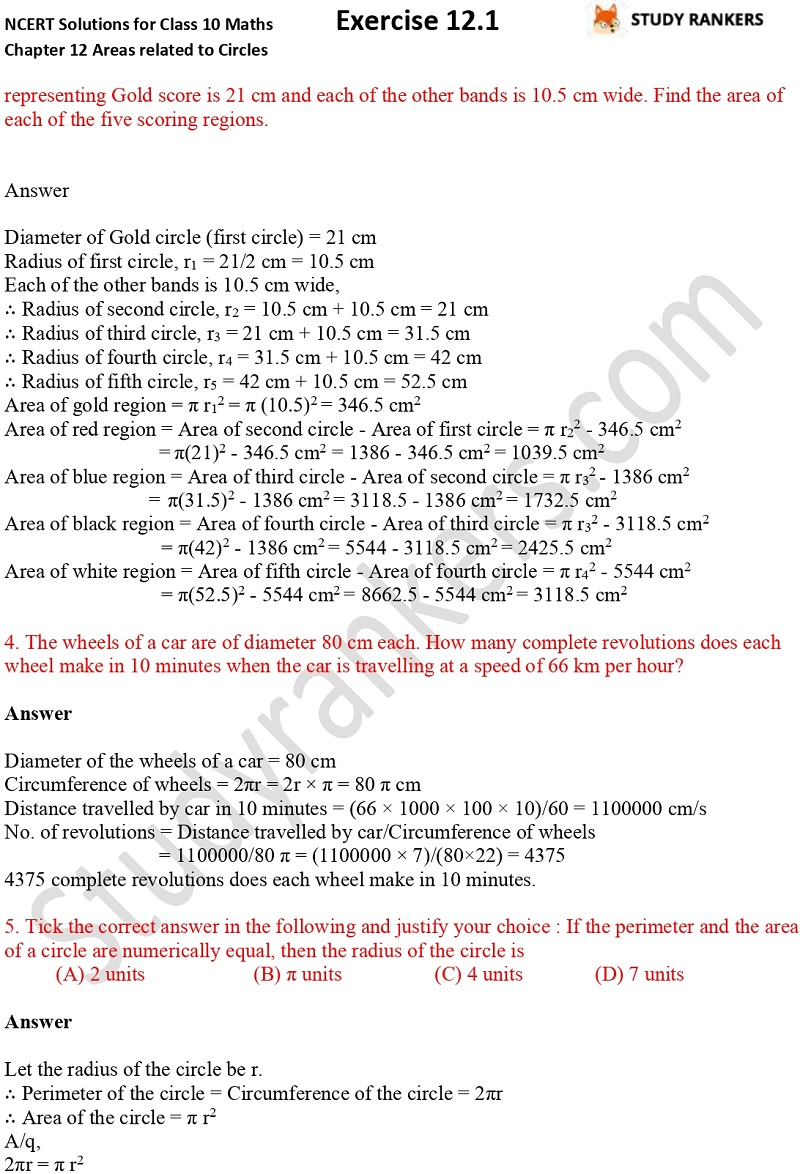>

## NCERT Solutions for Class 10 Maths Chapter 12 Areas Related to Circles Exercise 12.1

In this page, you will find Chapter 12 Areas related to Circles Exercise 12.1 Class 10 Maths NCERT Solutions that are very useful for completing your daily homework and at the same time understanding application of formula. A student must solve all these questions before moving further for advance books of Maths. Class 10 Maths NCERT Solutions provided here are prepared by Studyrankers experts which are detailed and accurate thus can help students in improving their marks in the examinations.

There are five questions in the exercise in which questions are related to finding area of circles such as the radii of two circles are 8 cm and 6 cm respectively. Find the radius of the circle having area equal to the sum of the areas of the two circles.